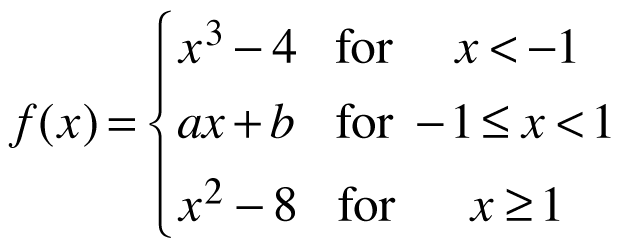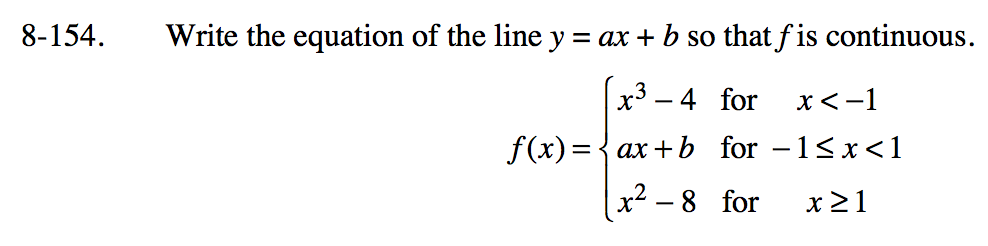### Home > PC3 > Chapter 8 > Lesson 8.3.5 > Problem8-154

8-154.
1. Write the equation of the line y = ax + b so that f is continuous. Homework Help ✎

2.When x = − 1, x3 − 4 = ax + b.

When x = 1, ax + b = x2 − 8.

(−1)3 − 4 = a(−1) + b

a(1) + b = (1)2 − 8